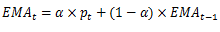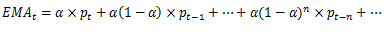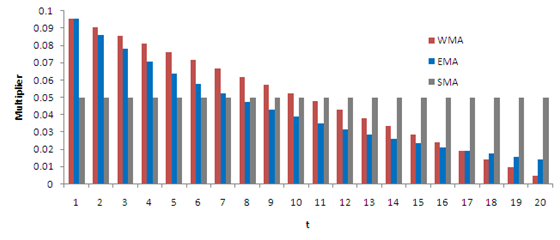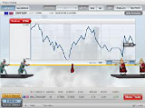# Exponential Moving Average (EMA)

EMA shortens the lag seen in SMA by giving more weight to recent prices, where the weight applied to the most recent price also depends on the number of periods in the moving average. There are three steps to calculating an exponential moving average. First, calculate the simple moving average which will be used as the previous period's EMA in the first calculation. Second, calculate the weighting multiplier. Third, calculate the exponential moving average. The formula below is for a 10-day EMA.

SMA: 10 period sum / 10

Multiplier: (2 / (Time periods + 1) ) = (2 / (10 + 1) ) = 0.1818 (18.18%)

EMA: {Close - EMA(previous day)} x multiplier + EMA(previous day).

A 10-period exponential moving average applies an 18.18% weighting to the most recent price. A 10-period EMA can also be called an 18.18% EMA. A 20-period EMA applies a 9.52% weighing to the most recent price (2/(20+1) = .0952). Notice that the weighting for the shorter time period is more than the weighting for the longer time period. In fact, the weighting drops by half every time the moving average period doubles.

For those who are interested in the details, the formula for EMA isorThe multiplier for the latest price is the same as in WMA. It drops exponentially but never reaches zero. Therefore all historical prices are factored in current time EMA calculation, although old prices carry very small weight. EMA address both criticisms suffered by SMA.### Forex Educational Articles & News

FeaturedTop 5 factors that affect exchange rates ...

There are many factors that affect exchange rates of currencies. However some are more important in currency trading than others. These are; Interest and Inflation rates, Trade balance, Currency market speculation, Foreign investment and Central bank market intervention. Learn how to use these factors in your forex tra ...Open a forex demo accountBest forex trading signals exp ...
Latest
Advertisement